Math Calculators, Lessons and Formulas

It is time to solve your math problem

mathportal.org

# Matrix calculator that shows work

This solver performs operations with matrices i.e. multiplication, addition and subtraction. The calculator will generate a step by step explanation for each of these operations.

Matrix operations calculator
Calculator will show work for each operation.
show help ↓↓ examples ↓↓
Matrix A:
Matrix B:
 Choose an operation
working...
examples
example 1:ex 1:
Multiply : $\left[ \begin{array}{cc} -3 & 2 \\ -5 & 4 \end{array} \right] \cdot \left[ \begin{array}{cc} -\frac{1}{2} & -3 \\ -6 & \frac{4}{3} \end{array} \right]$
example 2:ex 2:
Multiply : $\left[ \begin{array}{cc} 4 & 2 & -2 \\ 3 & 8 & 6 \end{array} \right] \cdot \left[ \begin{array}{cc} 2 & 6 \\ 3 & -1 \\ -5 & 11 \end{array} \right]$
example 3:ex 3:
$$\left[ \begin{array}{cc} 4 & \frac{2}{3} & -2 & \frac{1}{2} \\ -3 & 4 & -3 & \frac{3}{2} \\ 5 & 1 & -3 & 7 \\ \frac{1}{4} & 5 & 1 & 6 \end{array} \right] \cdot \left[ \begin{array}{cc} 5 & 2 & -\frac{5}{2} & 4 \\ 5 & 1 & 1 & 0 \\ 2 & 0 & 1 & -1 \\ 0 & -3 & 4 & 1 \end{array} \right]$$

## How to input matrix ?

### 1: Input matrix starting from the upper left-hand corner.

 Example: To input matrix: $\left[ \begin{array}{cc} -7 & 1/4 \\ -1.3 & -2/5 \end{array} \right]$ type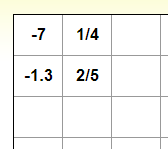### 2: You don't need to enter zeros.

 Example: To input matrix: $\left[ \begin{array}{ccc} 0 & 1 & 0 \\ -1 & 0 & 0 \\ 0 & 0 & 2/3 \end{array} \right]$ type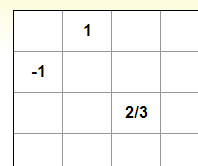### 3: You can copy and paste matrix from excel in 3 steps.

 Step 1: Copy matrix from excel Step 2: Select upper right cell Step 3: Press Ctrl+V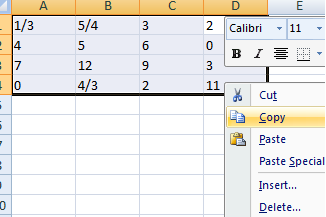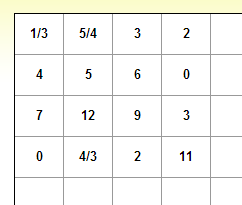### 5: To delete matrix

 Select whole matrix and click delete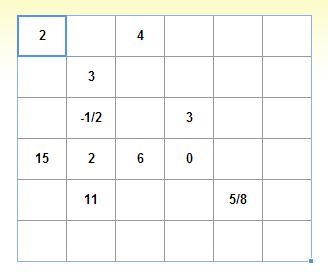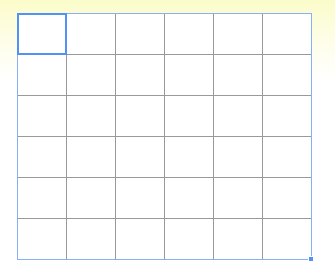Search our database of more than 200 calculators